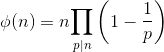Open in App
Not now

# UGC-NET | NTA UGC NET 2019 June – II | Question 23

• Last Updated : 03 Dec, 2021

Consider the Euler’s phi function given by

ϕ(n)=nΠp/n(1–1p)
where p runs over all the primes dividing n. What is the value of ϕ(45)?
(A) 3
(B) 12
(C) 6
(D) 24

Explanation: Euler’s Totient function =Where p=all prime factors of n
Now given n=45
Then prime factors of 45=3,5
ϕ(45)=45×(1−13)×(1−15)=24

So option 4 is correct.

Quiz of this Question
Please comment below if you find anything wrong in the above post

My Personal Notes arrow_drop_up
Related Articles